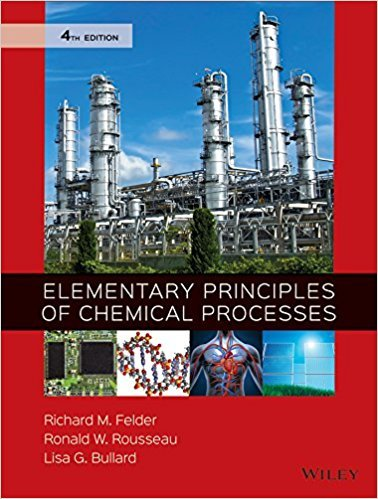×
×

# A distillation column is a process unit in which a feed mixture is separated by multipleISBN: 9780470616291 248

## Solution for problem 4.8 Chapter 4.17

Elementary Principles of Chemical Processes | 4th Edition

• Textbook Solutions
• 2901 Step-by-step solutions solved by professors and subject experts
• Get 24/7 help from StudySoup virtual teaching assistantsElementary Principles of Chemical Processes | 4th Edition

4 5 1 369 Reviews
20
5
Problem 4.8

A distillation column is a process unit in which a feed mixture is separated by multiple partial vaporizations and condensations to form two or more product streams. The overhead product stream is rich in the most volatile components of the feed mixture (the ones that vaporize most readily), and the bottom product stream is rich in the least volatile components.The following flowchart shows a distillation column with two feed streams and three product streams: m1(kg/h) 0.03 kg B/kg 0.97 kg C/kg Stream 1 Stream 3 5300 kg/h x2A(kg A/kg) x2B(kg B/kg) 1200 kg/h 0.70 kg A/kg x4B(kg B/kg) x4C(kg C/kg) m5(kg/h) 0.60 kg B/kg 0.40 kg C/kg m3(kg A/h) Stream 4 Stream 5 Stream 2 (a) How many independent material balances may be written for this system? (b) How many of the unknown flow rates and/or mole fractions must be specified before the others may be calculated? (See Example 4.3-4. Also, remember what you know about the component mole fractions of a mixturefor example, the relationship between x2A and x2B.) Briefly explain your answer. (c) Suppose values are given for m_ 1 and x2A. Give a series of equations, each involving only a single unknown, for the remaining variables. Circle the variable for which you would solve. (Once a variable has been calculated in one of these equations, it may appear in subsequent equations without being counted as an unknown.)

Step-by-Step Solution:
Step 1 of 3

GENERAL CHEMISTRY I – DR. STREIT Week of February 21, 2016 February 23, 2016 Ionic Bonding  Electrostatic attraction that holds oppositely charged ions together in an ionic compound (salts) o Transfer of electrons o Generally a metal with a nonmetal o Gain or lose electrons to fill an octet o Form large crystalline...

Step 2 of 3

Step 3 of 3

##### ISBN: 9780470616291

The answer to “A distillation column is a process unit in which a feed mixture is separated by multiple partial vaporizations and condensations to form two or more product streams. The overhead product stream is rich in the most volatile components of the feed mixture (the ones that vaporize most readily), and the bottom product stream is rich in the least volatile components.The following flowchart shows a distillation column with two feed streams and three product streams: m1(kg/h) 0.03 kg B/kg 0.97 kg C/kg Stream 1 Stream 3 5300 kg/h x2A(kg A/kg) x2B(kg B/kg) 1200 kg/h 0.70 kg A/kg x4B(kg B/kg) x4C(kg C/kg) m5(kg/h) 0.60 kg B/kg 0.40 kg C/kg m3(kg A/h) Stream 4 Stream 5 Stream 2 (a) How many independent material balances may be written for this system? (b) How many of the unknown flow rates and/or mole fractions must be specified before the others may be calculated? (See Example 4.3-4. Also, remember what you know about the component mole fractions of a mixturefor example, the relationship between x2A and x2B.) Briefly explain your answer. (c) Suppose values are given for m_ 1 and x2A. Give a series of equations, each involving only a single unknown, for the remaining variables. Circle the variable for which you would solve. (Once a variable has been calculated in one of these equations, it may appear in subsequent equations without being counted as an unknown.)” is broken down into a number of easy to follow steps, and 230 words. This textbook survival guide was created for the textbook: Elementary Principles of Chemical Processes, edition: 4. Elementary Principles of Chemical Processes was written by and is associated to the ISBN: 9780470616291. Since the solution to 4.8 from 4.17 chapter was answered, more than 224 students have viewed the full step-by-step answer. The full step-by-step solution to problem: 4.8 from chapter: 4.17 was answered by , our top Chemistry solution expert on 01/18/18, 04:13PM. This full solution covers the following key subjects: . This expansive textbook survival guide covers 204 chapters, and 1037 solutions.

Unlock Textbook Solution